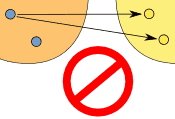Page

# Functions

## A Function is Special

A function is a type of equation.

A function has special rules it must follow:

In a function there can ONLY be ONE output(y) for every input (x).

Just like I stated before, a Function is Special! 🙂

• It must work for every possible input value
• And it has only one relationship for each input valueTHIS IS A FUNCTION!                  THIS IS NOT A FUNCTION!

### Example: y = x²

FUNCTIONCould also be written as a table:

X: x Y: x2
3 9
1 1
0 0
4 16
-4 16

It is a function, because:

• Every element in X is related to Y
• No element in X has two or more relationships
• The X values DO NOT repeat

So it follows the rules.

(Notice how both 4 and -4 relate to 16, which is allowed since 4 and -4 are two different numbers.)

### Example: This relationship is not a function:

NOT A FUNCTIONThis is not a function, for these reasons:

• Value “3” in X has no Y
• Value “4” in X has no Y
• Value “5” is related to more than one value in Y
• REMEMBER THE X VALUE CANNOT REPEAT

# Unit Rate

Unit rates are about the amount for 1 unit.

We use Ratio Tables to help solve for rates.
Our Ratio table looks like a tic-tac-toe table.We MUST label our table to know where to put our information

Ex. Bob drives 100 miles in 5 hours. How many miles does he travel every hour?Now we fill in the information that is given.

Bob drives 100 miles in 5 hours. How many miles does he travel every hour?Once we have entered what was given we need to see what the question is asking us.

Ex. Bob drives 100 miles in 5 hours. How many miles does he travel every hour?

Ask yourself, is the problem asking how many hours it takes for 1 mile or how many miles for 1 hour???????

If you said how many miles for 1 hour, you are correct!

So now we enter that into our Ratio Table.The x is the unknown. This is what we are solving for.To solve for x, we multiply 100 and 1. Then divide by the number left over which is 5.

We end up with x = 20.

So Bob drives 20 miles per hour.

Click here to watch a video with a different way to find unit rate.

Test yourself on unit rates here!